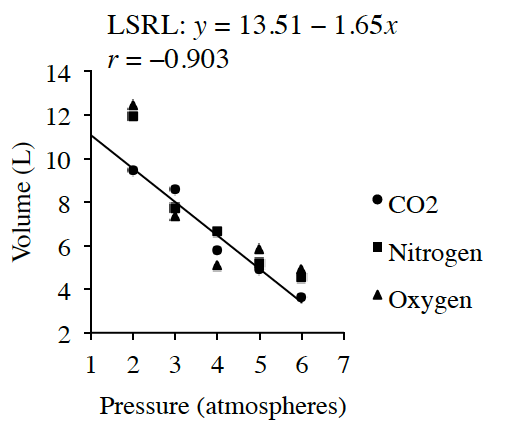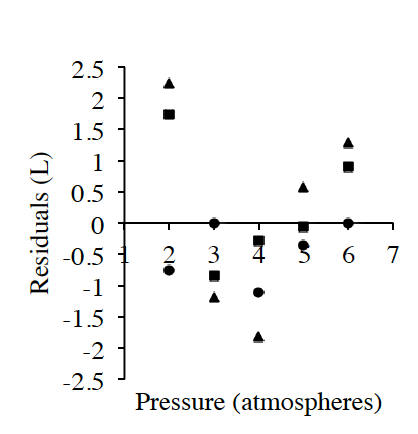### Home > GB8I > Chapter 8 Unit 9 > Lesson INT1: 8.1.1 > Problem8-16

8-16.

Brett is working in the chemistry lab trying to determine if he can predict the volume of three different gases (nitrogen, oxygen, and carbon dioxide) based upon the pressure applied to them. He kept all other characteristics of the gases constant. Brett performed the following analysis:Desmos GraphDesmos Residuals Graph

Volume

Pressure

Nitrogen

Oxygen

CO2

$2$

$11.94$

$12.45$

$9.45$

$3$

$7.71$

$7.37$

$8.56$

$4$

$6.62$

$5.09$

$5.79$

$5$

$5.2$

$5.83$

$4.9$

$6$

$4.51$

$4.9$

$3.61$

1. Describe the association, including the slope of the $LSRL$ and $R$-squared.

Is the association weak or strong?
Is the association negative or positive?
Are there any outliers?

Calculate $R^2$ (note that $r$ is given). Then comment on the variability in the data.

1. What is the residual with the greatest magnitude and what point does it belong to?

Refer to the right graph in the problem that has do with residuals.

The largest residual with the greatest magnitude value is about $2.3$ liters and it belongs to oxygen at $2$ atmospheres of pressure.

2. Using the $LSRL$ model, estimate the volume of a gas at $2.5$, $4.5$, and $6.5$ atmospheres. Use an appropriate precision.

Substitute the three values given in the problem into the $LSRL$ equation for $x$.

$9.40$ liters, $6.10$ liters, and $2.82$ liters

3. How well would this linear model work in predicting more extreme pressures? Support your answer.

Does the residual plot have random scatter, or is there a pattern?

What will the linear model predict when the pressure is greater than $8$ atmospheres?